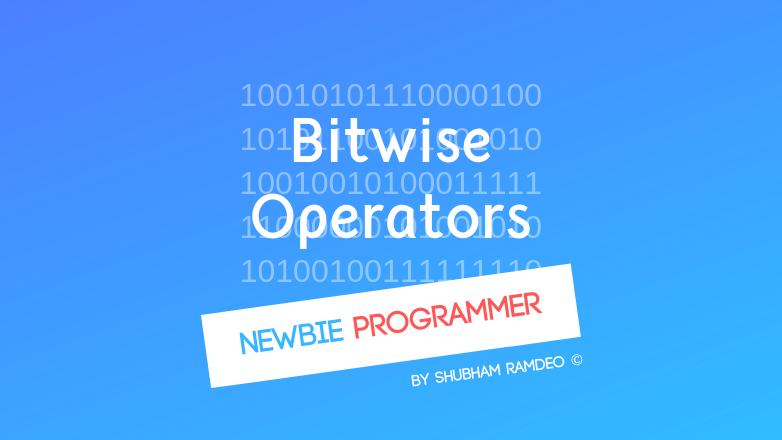# ShubhamRamdeo

September 8, 2015

# Bitwise Operators

Zeros and ones ! Bits ! They can be shifted and played with too using the Bitwise Operators !Welcome to the Newbie Programmer Series. We were discussing different types of operators and Bitwise Operators are of them. But Before I started writing this, I need to make some pre-required posts so that I can make Bitwise Operators easy to understand. We will discuss all of them now below. If you are new to this series, please go to index (click here) and read out all the previous articles so that you can easily understand what is going on below.

Bitwise Operators are very useful but their applications are very complex. I am not going to any deep detail.

This post will only help you to get a little introduction of them, we will not study deeply, just to introduce ourselves what they are.

Before Going into Bitwise Operators, Please checkout :

Now let’s get started.

The bitwise Operators are used to perform operations on the Bits. Yes those zeros and ones. By using bitwise operators, we can manipulate the ones and zeros of a value.

For example we have discussed many times the OR operators. It give ‘1’ in result when there exist at least one ‘1’. So on performing :

``````     110000101
OR   110100011
----------------------
ans. 110100111
``````

But before discussing them let make some variables :

``````A = 0011 1100 = 60
B = 0000 1101 = 13
``````

(These above variables are suggested by a friend of mine, not my idea ) If you have read the Binary Number System post linked above, You can easily get what zeros and ones would mean.

If you have read the Mathematical Reasoning post linked above, You can easily get what the following operators Below would mean :

### Binary AND operator ( & )

If both bits are 1 then resulting bit is 1 otherwise 0

So here: A&B =

``````     0011 1100
AND  0000 1101
--------------
ans. 0000 1100
``````

which gives (A&B) = 13 as 00001100 means 13 in Binary.

### Binary OR operator ( | )

If at least one bit is 1 then resulting bit is 1. Only when both bits are 0 then resulting bit is 0.

 So here: A B =
``````     0011 1100
OR   0000 1101
--------------
ans. 0011 1101
``````
 which gives (A B) = 61

### Binary XOR operator ( ^ )

Resulting bit is ‘1’ only if it is set in one of the operand but not both.

So here: A^B =

``````     0011 1100
^    0000 1101
--------------
ans. 0011 0001
``````

which gives (A^B) = 49

### Binary NOT ( or complement ) operator ( ~ )

Its simple gives complement of the bits. It reverses ones to zeros and zeros to one.

So here ( ~ A ) = 1100 0011 = -61

### Binary Left Shift Operator ( « )

The values of the bits are shifted left by the number of specified shifts. So for example,

``````A = 0011 1100
A >> 2 = 1111 0000
``````

All the bits got left by 2 spaces.

### Binary Right Shift Operators ( » )

The values of the bits are shifted right by the number of specified shifts. So for example,

``````A = 0011 1100
A << 2 = 0000 1111
``````

### Using with assignment

Remember the following shortcuts :

• C &= 2 is same as C = C & 2
•  C = 2 is same as C = C 2
• C ^= 2 is same as C = C ^ 2
• C «= 2 is same as C = C « 2
• C »= 2 is same as C = C » 2

That’s it for today. I will do NO programs for now as these things are already complex but we need to do an intro. I am gonna end operators with next post.

So Stay Connected.

#### You might also like:

(prev)
(next)

(rand) Creating your first HTML webpage
(rand) How technology affected our Lives? Happy being Busy?
(rand) What is happiness? What a trip with my friends taught me.